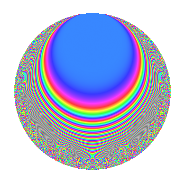# Properties

 Label 19.4.eLevel 19 Weight 4 Character orbit e Rep. character $$\chi_{19}(4,\cdot)$$ Character field $$\Q(\zeta_{9})$$ Dimension 24 Newform subspaces 1 Sturm bound 6 Trace bound 0

# Related objects

## Defining parameters

 Level: $$N$$ = $$19$$ Weight: $$k$$ = $$4$$ Character orbit: $$[\chi]$$ = 19.e (of order $$9$$ and degree $$6$$) Character conductor: $$\operatorname{cond}(\chi)$$ = $$19$$ Character field: $$\Q(\zeta_{9})$$ Newform subspaces: $$1$$ Sturm bound: $$6$$ Trace bound: $$0$$

## Dimensions

The following table gives the dimensions of various subspaces of $$M_{4}(19, [\chi])$$.

Total New Old
Modular forms 36 36 0
Cusp forms 24 24 0
Eisenstein series 12 12 0

## Trace form

 $$24q - 6q^{2} - 3q^{3} - 24q^{4} - 6q^{5} + 42q^{6} + 3q^{7} - 75q^{8} - 51q^{9} + O(q^{10})$$ $$24q - 6q^{2} - 3q^{3} - 24q^{4} - 6q^{5} + 42q^{6} + 3q^{7} - 75q^{8} - 51q^{9} + 75q^{10} + 39q^{11} - 219q^{12} - 156q^{13} + 93q^{14} - 192q^{15} + 504q^{16} + 12q^{17} + 264q^{18} + 546q^{19} - 198q^{20} + 453q^{21} - 6q^{22} + 6q^{23} + 192q^{24} - 498q^{25} - 639q^{26} - 870q^{27} - 1368q^{28} - 630q^{29} - 522q^{30} - 591q^{31} + 147q^{32} + 1506q^{33} - 408q^{34} + 2001q^{35} + 1059q^{36} - 72q^{37} + 2934q^{38} + 336q^{39} + 2886q^{40} - 477q^{41} + 237q^{42} + 588q^{43} - 3423q^{44} - 1569q^{45} - 1728q^{46} - 1242q^{47} - 4599q^{48} - 639q^{49} - 1788q^{50} + 9q^{51} + 2733q^{52} - 300q^{53} + 3777q^{54} + 315q^{55} + 4638q^{56} + 3342q^{57} - 2820q^{58} + 2097q^{59} + 1116q^{60} - 2316q^{61} - 1320q^{62} - 2979q^{63} - 1785q^{64} - 2433q^{65} - 1590q^{66} + 57q^{67} - 438q^{68} - 1767q^{69} - 213q^{70} - 792q^{71} - 1686q^{72} + 4068q^{73} + 4287q^{74} + 1332q^{75} + 5538q^{76} + 3786q^{77} + 2121q^{78} + 1824q^{79} - 2739q^{80} + 1536q^{81} + 2205q^{82} + 1071q^{83} - 1437q^{84} - 2394q^{85} - 5256q^{86} + 759q^{87} + 1101q^{88} - 3006q^{89} - 3822q^{90} - 3285q^{91} - 1452q^{92} - 135q^{93} - 1086q^{94} - 3078q^{95} - 1590q^{96} - 2535q^{97} - 2403q^{98} + 492q^{99} + O(q^{100})$$

## Decomposition of $$S_{4}^{\mathrm{new}}(19, [\chi])$$ into newform subspaces

Label Dim. $$A$$ Field CM Traces $q$-expansion
$$a_2$$ $$a_3$$ $$a_5$$ $$a_7$$
19.4.e.a $$24$$ $$1.121$$ None $$-6$$ $$-3$$ $$-6$$ $$3$$